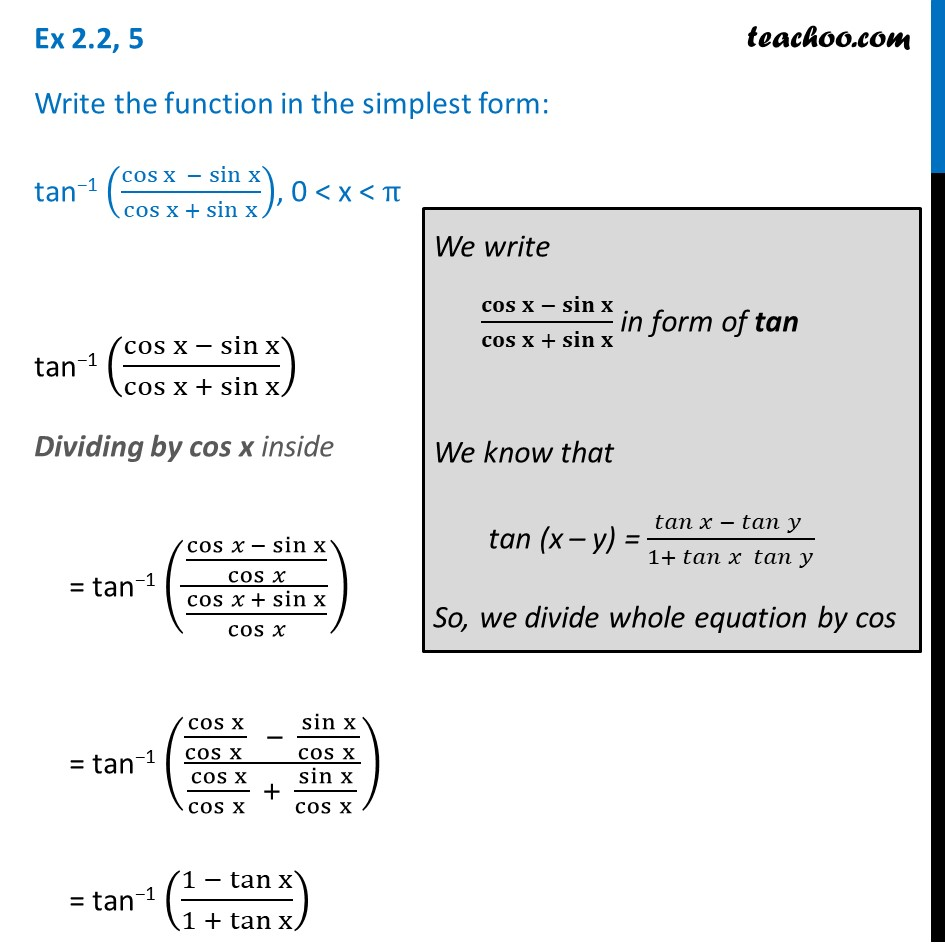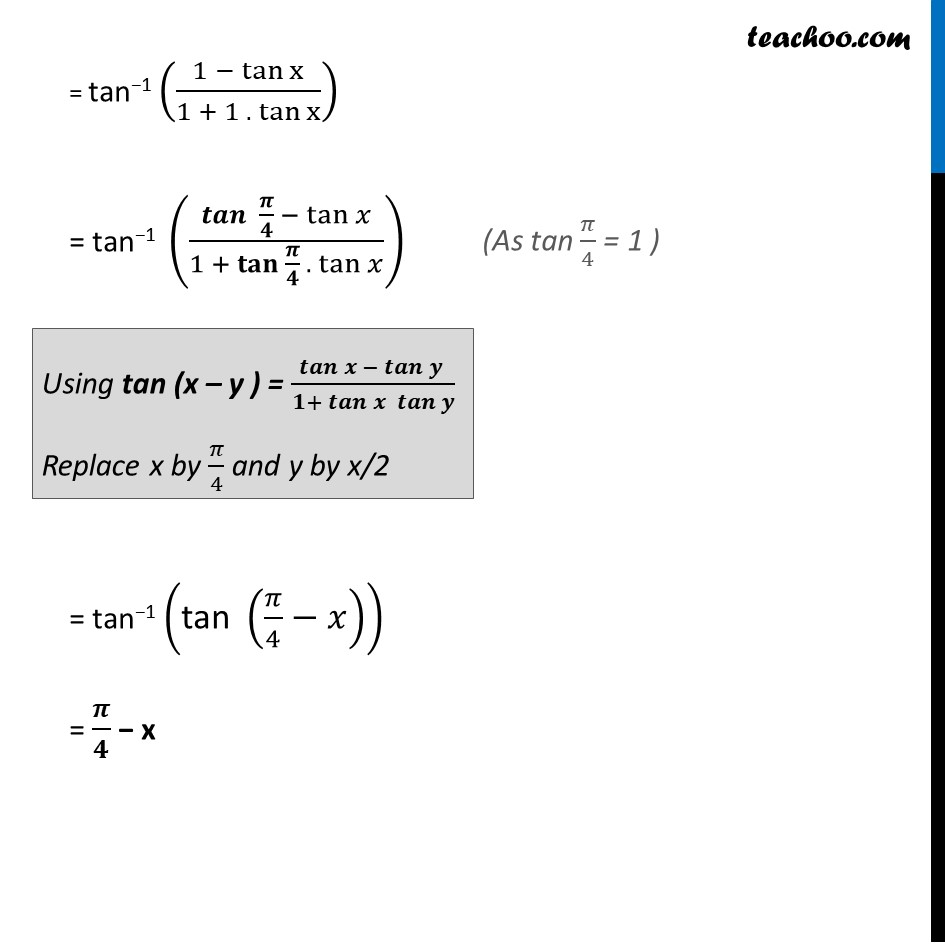Ex 2.2

Chapter 2 Class 12 Inverse Trigonometric Functions
Serial order wiseLearn in your speed, with individual attention - Teachoo Maths 1-on-1 Class

### Transcript

Ex 2.2, 5 Write the function in the simplest form: tan−1 (cos⁡〖x − sin⁡x 〗/cos⁡〖x + sin⁡x 〗 ), 0 < x < π tan−1 (cos⁡〖x − sin⁡x 〗/cos⁡〖x + sin⁡x 〗 ) Dividing by cos x inside = tan−1 (((cos⁡𝑥 − sin⁡x)/cos⁡𝑥 )/((cos⁡𝑥 + sin⁡x)/cos⁡𝑥 )) = tan−1 (((cos x)/cos⁡〖x 〗 − (sin x)/cos⁡〖x 〗 )/((cos x)/cos⁡〖x 〗 + (sin x)/cos⁡〖x 〗 )) = tan−1 ((1 − tan⁡x)/(1 +〖 tan〗⁡x )) We write 𝐜𝐨𝐬⁡〖𝐱 − 𝐬𝐢𝐧⁡𝐱 〗/𝐜𝐨𝐬⁡〖𝐱 + 𝐬𝐢𝐧⁡𝐱 〗 in form of tan We know that tan (x – y) = 𝑡𝑎𝑛⁡〖𝑥 −〖 𝑡𝑎𝑛〗⁡〖𝑦 〗 〗/(1+ 𝑡𝑎𝑛⁡〖𝑥 𝑡𝑎𝑛⁡𝑦 〗 ) So, we divide whole equation by cos = tan−1 ((1 − tan⁡x)/(1 +〖 1 . tan〗⁡x )) = tan−1 ((𝒕𝒂𝒏⁡〖 𝝅/𝟒〗 − tan⁡𝑥)/(1 + 〖𝐭𝐚𝐧 〗⁡〖𝝅/𝟒 .〖 tan〗⁡𝑥 〗 )) = tan−1 ("tan " (𝜋/4−𝑥)) = 𝝅/𝟒 − x Using tan (x – y ) = 𝒕𝒂𝒏⁡〖𝒙 −〖 𝒕𝒂𝒏〗⁡〖𝒚 〗 〗/(𝟏+ 𝒕𝒂𝒏⁡〖𝒙 𝒕𝒂𝒏⁡𝒚 〗 ) Replace x by 𝜋/4 and y by x/2